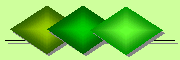De Claron LtdExpress Accountancy services at affordable prices
 Accounting Property Investment Reporting Company Secretarial ServicesHelp

Gross Potential Income

Gross Potential Income (GPI) on real estate is realised if a property is fully occupied and all rents are collected. To calculate the GPI one takes the number of rental units of property multiplied by the annual rent for each unit to obtain a total.

For example: An block of flats has six units. Three rent for \$1,000 per month and the other three rent for \$500 per month. The GPI is calculated as follows:

• 3 units x \$1,000 per month = \$3,000
• \$3,000 x 12 months = \$36,000
• 3 units x \$500 per month = \$1,500
• \$1,500 x 12 months = \$18,000
• \$36,000 + \$18,800 = \$54,000 in GPI